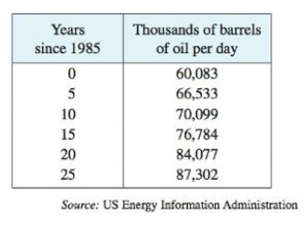Chapter 2.7, Problem 49E

Chapter
Section
Textbook Problem

The table shows world average daily oil consumption from 1985 to 2010 measured in thousands of barrels per day.(a) Compute the interpret the average rate of change from 1990 to 2005. What are its units?(b) Estimate the instantaneous rate of change in 2000 by taking the average of two average rates of change. What are its units?(a)

To determine

To find: The average rate of change from 1990 to 2005 and state the interpretation. Then describe the units for the average rate of change ΔCΔt.

Explanation

Given:

Let C(t) be the average daily consumption of oil measured in thousands of barrel per day with respect to time, where t is in years.

The table of average daily consumption of oil (in thousands of barrel per day) with respect to time t is given below.

 t (in years) C(t) (in thousands of barrels per day) 1985 60,083 1990 66,533 1995 70,099 2000 76,784 2005 84,077 2010 87,302

Formula used:

The average rate of change of C with respect to t on the interval [t1,t2] is,

ΔCΔt=C(t2)C(t1)t2t1 (1)

Calculation:

Obtain the average rate of change of C(t) from 1990 to 2005

(b)

To determine

To estimate: The instantaneous rate of change of C(t) in t=2000 and find its units.

Still sussing out bartleby?

Check out a sample textbook solution.

See a sample solution

The Solution to Your Study Problems

Bartleby provides explanations to thousands of textbook problems written by our experts, many with advanced degrees!

Get Started

Find f. f(t) = t + 1/t3, t 0, f(1) = 6

Single Variable Calculus: Early Transcendentals, Volume I

Find the mean, median, and mode for the following scores; 8, 7, 5, 7, 0, 10, 2, 4, 11, 7, 8, 7

Essentials of Statistics for The Behavioral Sciences (MindTap Course List)

Give the domain of each function in Problems 1-8.

Mathematical Applications for the Management, Life, and Social Sciences

. ∞ −∞ 1 −1

Study Guide for Stewart's Single Variable Calculus: Early Transcendentals, 8th